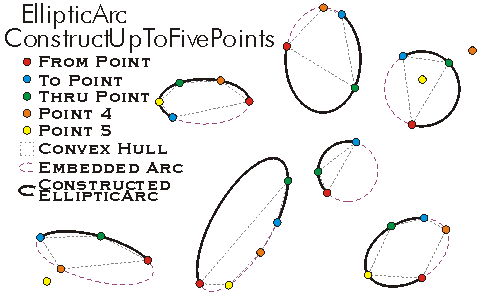This document is archived and information here might be outdated.  Recommended version.

IConstructEllipticArc.ConstructUpToFivePoints Method (ArcObjects .NET 10.7 SDK)
 ArcObjects Help for .NET developers > ArcObjects Help for .NET developers > ArcObjects namespaces > Geometry > ESRI.ArcGIS.Geometry > Interfaces > IC > IConstructEllipticArc Interface > IConstructEllipticArc.ConstructUpToFivePoints Method
 ArcGIS Developer Help

# IConstructEllipticArc.ConstructUpToFivePoints Method

Constructs an elliptic arc, given up to 5 points, such that the embedded ellipse passes through as many as possible. The arc will start at the first point and end at the second, passing through the third.

```[Visual Basic .NET]
Public Sub ConstructUpToFivePoints ( _
ByVal from As IPoint, _
ByVal to As IPoint, _
ByVal thru As IPoint, _
ByVal point4 As IPoint, _
ByVal point5 As IPoint _
)
```
```[C#]
public void ConstructUpToFivePoints (
IPoint from,
IPoint to,
IPoint thru,
IPoint point4,
IPoint point5
);
```
```[C++]
HRESULT ConstructUpToFivePoints(
IPoint* from,
IPoint* to,
IPoint* thru,
IPoint* point4,
IPoint* point5
);
```
```[C++]
Parameters
from
from is a parameter of type IPoint*
to
to is a parameter of type IPoint*
thru
thru is a parameter of type IPoint*
point4
point4 is a parameter of type IPoint*
point5
point5 is a parameter of type IPoint*
```

#### Product Availability

Available with ArcGIS Engine, ArcGIS Desktop, and ArcGIS Server.

#### Description

Constructs an EllipticArc using as many of the zero to five input points as possible.  The first input point is always the From Point of the constructed EllipticArc.  The second input point is always the To Point of the constructed EllipticArc.  The third point is the Thru Point of the EllipticArc and is always guaranteed to lie on the constructed EllipticArc.  The fourth and fifth points may lie on either the constructed EllipticArc or the embedded extension of the EllipticArc.  The first three input points are guaranteed to be used in the construction of the EllipticArc.  The fourth and fifth points are used in preferential order as long as they do not create an invalid EllipticArc.  All points used to construct an EllipticArc must lie on a Convex Hull, otherwise the fourth or fifth non-convex points are discarded to maintain convexity.  Zero input points defines an empty EllipticArc.  One input point defines a degenerate IsPoint EllipticArc.  Two disjoint input points uniquely define a degenerate IsLine EllipticArc with the points at the end of the semiMajor axis with a Minor/Major Ratio of 0.  Three non-colinear input points uniquely define an IsCircular EllipticArc in the same manner as ConstructThreePoints constructs a CircularArc.  Four input points on a convex hull may be sufficient to uniquely define an unrotated (or Pi / 2 rotated) EllipticArc.  For cases where an unrotated EllipticArc cannot be fit to the input points, a reasonable rotated EllipticArc is constructed to fit the input points (this EllipticArc is not the only rotated EllipticArc that can be fit to the input points.).

#### Remarks[C#]
```private void ConstructFivePointArc()
{
IPoint point1 = new PointClass();
point1.PutCoords(5, 10);
IPoint point2 = new PointClass();
point2.PutCoords(15, 10);
IPoint point3 = new PointClass();
point3.PutCoords(15, 100);
IPoint point4 = new PointClass();
point4.PutCoords(50, 10);
IPoint point5 = new PointClass();
point5.PutCoords(50, 100);
IConstructEllipticArc constructEllipticArc = new EllipticArcClass();
constructEllipticArc.ConstructUpToFivePoints(point1, point2, point3, point4, point5);
IEllipticArc ellipitcArc = constructEllipticArc as IEllipticArc;
String report = "X, Y Center Point : " + ellipitcArc.CenterPoint.X + " , " + ellipitcArc.CenterPoint.Y + "\n" +
"Center angle in rad : " + ellipitcArc.CentralAngle + "\n" +
"Length : " + ellipitcArc.Length;
System.Windows.Forms.MessageBox.Show(report);
}
```
[Visual Basic .NET]

Private Sub ConstructUpToFivePointsEllipticArc()
Dim pCons As ESRI.ArcGIS.Geometry.IConstructEllipticArc
Dim pTr As ESRI.ArcGIS.Geometry.IPoint
Dim pFr As ESRI.ArcGIS.Geometry.IPoint
Dim pTo As ESRI.ArcGIS.Geometry.IPoint
Dim p4 As ESRI.ArcGIS.Geometry.IPoint
Dim p5 As ESRI.ArcGIS.Geometry.IPoint
Dim pEArc As ESRI.ArcGIS.Geometry.IEllipticArc
pTr = New ESRI.ArcGIS.Geometry.Point
pFr = New ESRI.ArcGIS.Geometry.Point
pTo = New ESRI.ArcGIS.Geometry.Point
p4 = New ESRI.ArcGIS.Geometry.Point
p5 = New ESRI.ArcGIS.Geometry.Point
pFr.PutCoords(0, -100)
pTo.PutCoords(0, -100)
pTr.PutCoords(200, 0)
p4.PutCoords(0, 100)
p5.PutCoords(-200, -0)
pCons = New ESRI.ArcGIS.Geometry.EllipticArc
pCons.ConstructUpToFivePoints(pFr, pTo, pTr, p4, p5)
pEArc = pCons 'QI
'Report
Debug.Print("X, Y Center Point : " & pEArc.CenterPoint.X & " , " & pEArc.CenterPoint.Y)
Debug.Print("Center angle in rad : " & pEArc.CentralAngle)
Debug.Print("Length : " & pEArc.Length)
End Sub